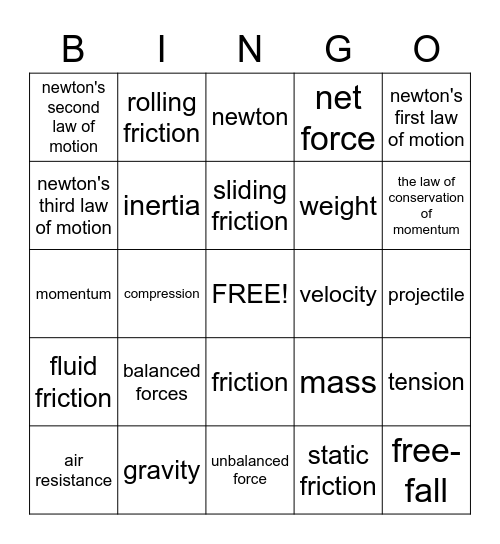Newtons Laws and MotionThis bingo card has a free space and 24 words: balanced forces, gravity, sliding friction, air resistance, newton's first law of motion, newton's second law of motion, newton's third law of motion, rolling friction, velocity, friction, weight, momentum, mass, tension, inertia, static friction, projectile, newton, the law of conservation of momentum, compression, free-fall, fluid friction, net force and unbalanced force.

Play Online